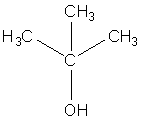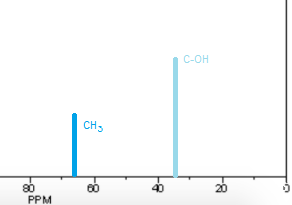# Construct a proton-decoupled C NMR spectrum for tert-butyl alcohol.

## Question:

Construct a proton-decoupled C NMR spectrum for tert-butyl alcohol.

## {eq}^1H {/eq}-Decoupled {eq}^{13}C {/eq}-NMR

A {eq}^1H {/eq}-Decoupled {eq}^{13}C {/eq}-NMR spectrum is one where the signals arising from different {eq}^{13}C {/eq} environment is not split, which otherwise would be split, if coupled, due to adjacent protons.

The structure of tert-butyl alcohol is shown below. We can see that there are only 2 carbon environment. One carbon is bonded to the hydroxyl group (OH), and 3 carbons are all bonded to this carbon with three protons i.e. they are all {eq}CH_3 {/eq} groups.Therefore, there will be 2 signals on a {eq}^{13}C {/eq}-NMR spectrum. Where these signals are, can be found on a chemical shift table. A C-OH signal is found in the region of 50-90 ppm. A {eq}CH_3 {/eq} group is usually found around 0-50 ppm. Because the {eq}CH_3 {/eq} groups are relatively close to the OH group, the signal will be seen at the higher end of the range. This is because oxygen is electronegative and so pushes electron density to itself. In turn, this deshields the carbon nucleus which 'feels' a larger magnetic field, pushing the peak downfield. A sketch of an approximate NMR spectrum is given below. Note that the integration for the peak downfield will be 3, and the other will show an integration of 1.